# Lab 8.1 Simple Loops

 Team-Fly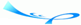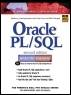Oracle® PL/SQL® Interactive Workbook, Second EditionBy Benjamin Rosenzweig, Elena Silvestrova Table of Contents Chapter 8.  Iterative Control

Lab Objectives

After this Lab, you will be able to:

• Use Simple Loops with EXIT Conditions

• Use Simple Loops with EXIT WHEN Conditions

A simple loop, as you can see from its name, is the most basic kind of loop and has the following structure:

` LOOP     STATEMENT 1;     STATEMENT 2;     …     STATEMENT N;  END LOOP; `

The reserved word LOOP marks the beginning of the simple loop. Statements 1 through N are a sequence of statements that is executed repeatedly. These statements consist of one or more of the standard programming structures. END LOOP is a reserved phrase that indicates the end of the loop construct.

The flow of logic from this structure is illustrated in Figure 8.1.

##### Figure 8.1. Simple Loop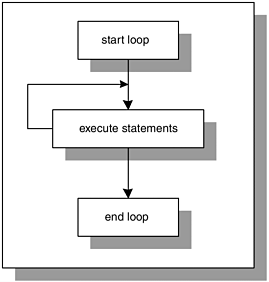Every time the loop is iterated, a sequence of statements is executed, and then control is passed back to the top of the loop. The sequence of statements will be executed an infinite number of times, because there is no statement specifying when the loop must terminate. Hence, a simple loop is called an infinite loop because there is no means to exit the loop. A properly constructed loop needs to have an exit condition that determines when the loop is complete. This exit condition has two forms: EXIT and EXIT WHEN.

#### EXIT

The EXIT statement causes a loop to terminate when the EXIT condition evaluates to TRUE. The EXIT condition is evaluated with the help of an IF statement. When the EXIT condition is evaluated to TRUE, control is passed to the first executable statement after the END LOOP statement. This is indicated by the following:

` LOOP     STATEMENT 1;     STATEMENT 2;     IF CONDITION THEN        EXIT;     END IF;  END LOOP;  STATEMENT 3; `

In this example, you can see that after the EXIT condition evaluates to TRUE, control is passed to STATEMENT 3, which is the first executable statement after the END LOOP statement.The EXIT statement is valid only when placed inside of a loop. When placed outside of a loop, it will cause a syntax error. To avoid this error, use the RETURN statement to terminate a PL/SQL block before its normal end is reached as follows:

` BEGIN     DBMS_OUTPUT.PUT_LINE ('Line 1');     RETURN;     DBMS_OUTPUT.PUT_LINE ('Line 2');  END; `

This example produces the output:

` Line 1  PL/SQL procedure successfully completed. `

Because the RETURN statement terminates the PL/SQL block, the second DBMS_OUTPUT.PUT_LINE statement is never executed.

#### EXIT WHEN

The EXIT WHEN statement causes a loop to terminate only if the EXIT WHEN condition evaluates to TRUE. Control is then passed to the first executable statement after the END LOOP statement. The structure of a loop using an EXIT WHEN clause is as follows:

` LOOP     STATEMENT 1;     STATEMENT 2;     EXIT WHEN CONDITION;  END LOOP;  STATEMENT 3; `

This flow of logic from the EXIT and EXIT WHEN statements is illustrated in Figure 8.2.

##### Figure 8.2. Simple Loop with the EXIT Condition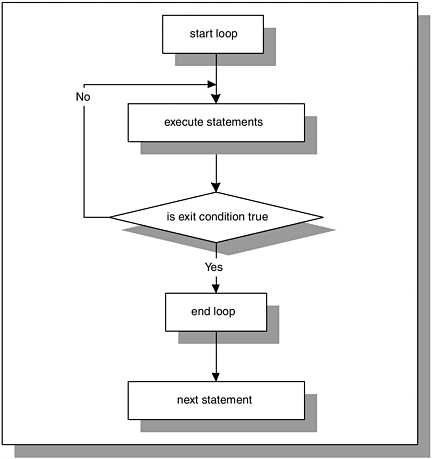Figure 8.2 shows that during each iteration, the loop executes a sequence of statements. Control is then passed to the EXIT condition of the loop. If the EXIT condition evaluates to FALSE, control is passed to the top of the loop. The sequence of statements will be executed repeatedly until the EXIT condition evaluates to TRUE. When the EXIT condition evaluates to TRUE, the loop is terminated, and control is passed to the next executable statement following the loop.

Figure 8.2 also shows that the EXIT condition is included in the body of the loop. Therefore, the decision about loop termination is made inside the body of the loop, and the body of the loop, or a part of it, will always be executed at least once. However, the number of iterations of the loop depends on the evaluation of the EXIT condition and is not known until the loop completes.

As mentioned earlier, Figure 8.2 illustrates that the flow of logic for the structure of EXIT and EXIT WHEN statements is the same even though two different forms of EXIT condition are used. In other words,

` IF CONDITION THEN     EXIT;  END IF; `

is equivalent to

` EXIT WHEN CONDITION; `It is important to note that when the EXIT statement is used without an EXIT condition, the simple loop will execute only once. Consider the following example.

` DECLARE     v_counter NUMBER := 0;  BEGIN     LOOP        DBMS_OUTPUT.PUT_LINE ('v_counter = '||v_counter);        EXIT;     END LOOP;  END; `

This example produces the following output:

` v_counter = 0  PL/SQL procedure successfully completed. `

Because the EXIT statement is used without an EXIT condition, the loop is terminated as soon as the EXIT statement is executed.

#### Lab 8.1 Exercises

##### 8.1.1 Use Simple Loops with EXIT Conditions

In this exercise, you will use the EXIT condition to terminate a simple loop, and a special variable, v_counter, which keeps count of the loop iterations. With each iteration of the loop, the value of v_counter will be incremented and displayed on the screen.

Create the following PL/SQL script:

` -- ch08_1a.sql, version 1.0  SET SERVEROUTPUT ON  DECLARE     v_counter BINARY_INTEGER := 0;  BEGIN     LOOP        -- increment loop counter by one        v_counter := v_counter + 1;        DBMS_OUTPUT.PUT_LINE ('v_counter = '||v_counter);        -- if EXIT condition yields TRUE exit the loop        IF v_counter = 5 THEN           EXIT;        END IF;     END LOOP;     -- control resumes here     DBMS_OUTPUT.PUT_LINE ('Done…');  END; `The statement

` v_counter := v_counter + 1 `

is used often when working with a loop. Variable v_counter is a loop counter that tracks the number of times the statements in the body of the loop are executed. You will notice that for each iteration of the loop, its value is incremented by 1. However, it is very important to initialize the variable v_counter for successful termination of the loop. If v_counter is not initialized, its value is NULL. Then, the statement

` v_counter := v_counter + 1 `

will never increment the value of v_counter by one, because NULL + 1 evaluates to NULL. As result, the EXIT condition will never yield TRUE, and the loop will become infinite.

Execute the script, and then answer the following questions.

 a) What output was printed on the screen? b) How many times was the loop executed? c) What is the EXIT condition for this loop? d) How many times will the value of the variable v_counter be displayed if the DBMS_OUTPUT.PUT_LINE statement is used after the END IF statement? e) Why does the number of times the loop counter value is displayed on the screen differ when the DBMS_OUTPUT.PUT_ LINE statement is placed after the END IF statement? f) Rewrite this script using the EXIT WHEN condition instead of the EXIT condition, so that it produces the same result.

##### 8.1.2 Use Simple Loops with EXIT WHEN Conditions

In this exercise, you will use the EXIT WHEN condition to terminate the loop. You will add a number of sections for a given course number. Try to answer the questions before you run the script. Once you have answered the questions, run the script and check your answers.

Create the following PL/SQL script:

` -- ch08_2a.sql, version 1.0  SET SERVEROUTPUT ON  DECLARE     v_course course.course_no%type := 430;     v_instructor_id instructor.instructor_id%type := 102;     v_sec_num section.section_no%type := 0;  BEGIN     LOOP        -- increment section number by one        v_sec_num := v_sec_num + 1;        INSERT INTO section           (section_id, course_no, section_no,           instructor_id, created_date, created_by,           modified_date, modified_by)        VALUES           (section_id_seq.nextval, v_course, v_sec_num,           v_instructor_id, SYSDATE, USER, SYSDATE,           USER);        -- if number of sections added is four exit the loop        EXIT WHEN v_sec_num = 4;     END LOOP;     -- control resumes here     COMMIT;  EXCEPTION     WHEN OTHERS THEN        DBMS_OUTPUT.PUT_LINE ('An error has occurred');  END; `

Notice that the INSERT statement contains an Oracle built-in function called USER. At first glance, this function looks like a variable that has not been declared. This function returns the name of the current user. In other words, it will return the login name that you use when connecting to Oracle.

Try to answer the following questions first, and then execute the script:

 a) How many sections will be added for the specified course number? b) How many times will the loop be executed if the course number is not valid? c) How would you change this script to add 10 sections for the specified course number? d) How would you change the script to add only even-numbered sections (maximum section number is 10) for the specified course number? e) How many times will the loop be executed in this case?

#### Lab 8.1 Exercise Answers

This section gives you some suggested answers to the questions in Lab 8.1, with discussion related to how those answers resulted. The most important thing to realize is whether your answer works. You should figure out the implications of the answers here and what the effects are from any different answers you may come up with.

##### 8.1.1 Answers

 a) What output was printed on the screen? A1: Answer: Your output should look like the following:` v_counter = 1 v_counter = 2 v_counter = 3 v_counter = 4 v_counter = 5 Done... PL/SQL procedure successfully completed. `Every time the loop is run, the statements in the body of the loop are executed. In this script, the value of v_counter is incremented by 1 and displayed on the screen. The EXIT condition is evaluated for each value of v_counter. Once the value of v_counter increases to 5, the loop is terminated. For the first iteration of the loop, the value of v_counter is equal to 1, and it is displayed on the screen, and so forth. After the loop has terminated, "Done..." is displayed on the screen. b) How many times was the loop executed? A2: Answer: The loop was executed five times.Once the value of v_counter increases to 5, the IF statement ` IF v_counter = 5 THEN EXIT; END IF; `evaluates to TRUE, and the loop is terminated.The loop counter tracks the number of times the loop is executed. You will notice that in this exercise, the maximum value of v_counter is equal to the number of times the loop is iterated. c) What is the EXIT condition for this loop? A3: Answer: The EXIT condition for this loop is v_counter = 5.The EXIT condition is used as a part of an IF statement. The IF statement evaluates the EXIT condition to TRUE or FALSE, based on the current value of v_counter. d) How many times will the value of the variable v_counter be displayed if the DBMS_OUTPUT.PUT_LINE statement is used after the END IF statement? A4: Answer: The value of v_counter will be displayed four times.` LOOP v_counter := v_counter + 1; IF v_counter = 5 THEN EXIT; END IF; DBMS_OUTPUT.PUT_LINE ('v_counter = '||v_counter); END LOOP; `Assume that the loop has iterated four times already. Then the value of v_counter is incremented by 1, so v_counter is equal to 5. Next, the IF statement evaluates the EXIT condition. The EXIT condition yields TRUE, and the loop is terminated. The DBMS_OUTPUT.PUT_LINE statement is not executed for the fifth iteration of the loop because control is passed to the next executable statement after the END LOOP statement. Thus, only four values of v_counter are displayed on the screen. e) Why does the number of times the loop counter value is displayed on the screen differ when the DBMS_OUTPUT.PUT_ LINE statement is placed after the END IF statement? A5: Answer: When the DBMS_OUTPUT.PUT_LINE statement is placed before the IF statement, the value of v_counter is displayed on the screen first. Then it is evaluated by the IF statement.The fifth iteration of the loop "v_counter = 5" is displayed first, then the EXIT condition yields TRUE and the loop is terminated.When the DBMS_OUTPUT.PUT_LINE statement is placed after the END IF statement, the EXIT condition is evaluated prior to the execution of the DBMS_OUTPUT.PUT_ LINE statement. Thus, for the fifth iteration of the loop, the EXIT condition evaluates to TRUE before the value of v_counter is displayed on the screen by the DBMS_OUTPUT.PUT_LINE statement. f) Rewrite this script using the EXIT WHEN condition instead of the EXIT condition, so that it produces the same result. A6: Answer: Your script should look similar to the following script. Changes are shown in bold letters.` -- ch08_1b.sql, version 2.0 SET SERVEROUTPUT ON DECLARE v_counter BINARY_INTEGER := 0; BEGIN LOOP -- increment loop counter by one v_counter := v_counter + 1; DBMS_OUTPUT.PUT_LINE ('v_counter = '||v_counter); -- if EXIT WHEN condition yields TRUE exit the loop EXIT WHEN v_counter = 5; END LOOP; -- control resumes here DBMS_OUTPUT.PUT_LINE ('Done…'); END; `Notice that the IF statement has been replaced by the EXIT WHEN statement. The rest of the statements in the body of the loop do not need to be changed.

##### 8.1.2 Answers

 a) How many sections will be added for the specified course number? A1: Answer: Four sections were added for the given course number. b) How many times will the loop be executed if the course number is not valid? A2: Answer: The loop will be executed one time.If the course number is not valid, the INSERT statement` INSERT INTO section (section_id, course_no, section_no, instructor_id, created_date, created_by, modified_date, modified_by) VALUES (section_id_seq.nextval, v_course, v_sec_num, v_instructor_id, SYSDATE, USER, SYSDATE, USER); `will cause an exception to be raised. As soon as an exception is raised, control is passed out of the loop to the exception handler. Therefore, if the course number is not valid, the loop will be executed only once. c) How would you change this script to add 10 sections for the specified course number? A3: Answer: Your script should look similar to the following script. Changes are shown in bold letters.` -- ch08_2b.sql, version 2.0 DECLARE v_course course.course_no%type := 430; v_instructor_id instructor.instructor_id%type := 102; v_sec_num section.section_no%type := 0; BEGIN LOOP -- increment section number by one v_sec_num := v_sec_num + 1; INSERT INTO section (section_id, course_no, section_no, instructor_id, created_date, created_by, modified_date, modified_by) VALUES (section_id_seq.nextval, v_course, v_sec_num, v_instructor_id, SYSDATE, USER, SYSDATE, USER); -- if number of sections added is ten exit the loop EXIT WHEN v_sec_num = 10; END LOOP; -- control resumes here COMMIT; EXCEPTION WHEN OTHERS THEN DBMS_OUTPUT.PUT_LINE ('An error has occurred'); END; `In order to add 10 sections for the given course number, the test value of v_sec_num in the EXIT condition is changed to 10.Note that before you execute this version of the script you need to delete records from the SECTION table that were added when you executed the original example. If you did not run the original script, you do not need to delete records from the SECTION table.The SECTION table has a unique constraint defined on the COURSE_NO and SECTION_NO columns. In other words, the combination of course and section numbers allows you to uniquely identify each row of the table. When the original script is executed, it creates four records in the SECTION table for course number 430, section numbers 1, 2, 3, and 4. When the new version of this script is executed, the unique constraint defined on the SECTION table is violated because there already are records corresponding to course number 430 and section numbers 1, 2, 3, and 4. Therefore, these rows must be deleted from the SECTION table as follows:` DELETE FROM section WHERE course_no = 430 AND section_no <= 4; `Once these records are deleted from the SECTION table, you can execute the new version of the script. d) How would you change the script to add only even-numbered sections (maximum section number is 10) for the specified course number? A4: Answer: Your script should look similar to the following script. Changes are shown in bold letters. In order to run this script, you will need to delete records from the SECTION table that were added by the previous version. With each iteration of the loop, the value of v_sec_num should be incremented by two, as shown:` -- ch08_2c.sql, version 3.0 SET SERVEROUTPUT ON DECLARE v_course course.course_no%type := 430; v_instructor_id instructor.instructor_id%type := 102; v_sec_num section.section_no%type := 0; BEGIN LOOP -- increment section number by two v_sec_num := v_sec_num + 2; INSERT INTO section (section_id, course_no, section_no, instructor_id, created_date, created_by, modified_date, modified_by) VALUES (section_id_seq.nextval, v_course, v_sec_num, v_instructor_id, SYSDATE, USER, SYSDATE, USER); -- if number of sections added is ten exit the loop EXIT WHEN v_sec_num = 10; END LOOP; -- control resumes here COMMIT; EXCEPTION WHEN OTHERS THEN DBMS_OUTPUT.PUT_LINE ('An error has occurred'); END; ` e) How many times will the loop be executed in this case? A5: Answer: The loop is executed five times when even-numbered sections are added for the given course number.

#### Lab 8.1 Self-Review Questions

In order to test your progress, you should be able to answer the following questions.

Answers appear in Appendix A, Section 8.1.

 1) How many times is a simple loop executed if there is no EXIT condition specified? _____ The loop does not execute at all._____ The loop executes once._____ The loop executes an infinite number of times. 2) How many times is a simple loop executed if the EXIT statement is used without an EXIT condition? _____ The loop does not execute at all._____ The loop executes once._____ The loop executes an infinite number of times. 3) What value must the EXIT condition evaluate to in order for the loop to terminate? _____ TRUE_____ FALSE_____ NULL 4) What statement must be executed before control can be passed from the body of the loop to the first executable statement outside of the loop? _____ LOOP statement_____ END LOOP statement_____ EXIT statement_____ RETURN statement 5) A simple loop will execute a minimum of which of the following? _____ Zero times_____ One time_____ Infinite number of times

 Team-FlyTop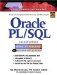Oracle PL/SQL Interactive Workbook (2nd Edition)
ISBN: 0130473200
EAN: 2147483647
Year: 2002
Pages: 146

Similar book on Amazon

flylib.com © 2008-2017.
If you may any questions please contact us: flylib@qtcs.net